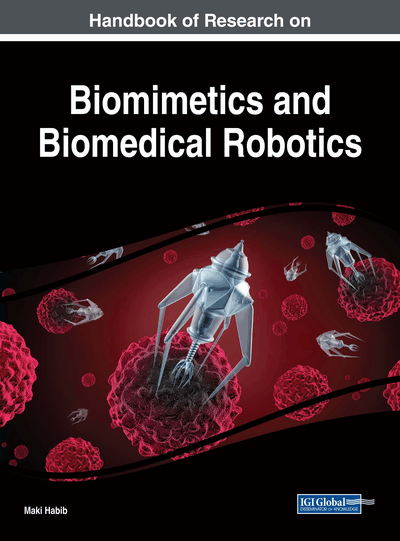# Neural Networks to Solve Nonlinear Inverse Kinematic Problems

Fusaomi Nataga (Tokyo University of Science, Japan), Maki K. Habib (The American University in Cairo, Egypt) and Keigo Watanabe (Okayama University, Japan)
DOI: 10.4018/978-1-5225-2993-4.ch009
Available
\$37.50
No Current Special Offers

## Abstract

In making neural networks learn nonlinear relations effectively, it is desired to have appropriate training sets. In the proposed method, after a certain number of iterations, input-output pairs having worse errors are extracted from the original training set and form a new temporary set. From the following iteration, the temporary set is applied to the neural networks instead of the original set. In this case, only pairs with worse errors are used for updating the weights until the mean value of errors decreases to a desired level. Once the learning is conducted using the temporary set, the original set is applied again instead of the temporary set. The effectiveness of the proposed approach is demonstrated through simulations using kinematic models of a leg module with a serial link structure and an industrial robot.
Chapter Preview
Top

## Introduction

For example, when designing a leg module with a serial link structure as shown in Fig. 1 for a multi-legged robot used in mechatronics education, first of all, its inverse kinematics problem must be solved. Up to now, biomimetic artificial neural network approaches have been devoted to robotic inverse kinematics problems. The neural network technique mimicking the human grain is known as one of the important results in biomedical engineering. In this first section, several related researches that challenged to solve inverse kinematics problems of manipulators or mechatronics systems are surveyed. Mao and Hsia proposed a neural network approach to solve the inverse kinematics of redundant robot manipulators in an environment with obstacles (Mao 1997), in which only the knowledge of the robot forward kinematics functions was required and the neural network was trained in the inverse modeling manner. Tejomurtula and Kak (1999) proposed a solution of inverse kinematics concerning a simple two link manipulator (Tejomurtula 1999). It was reported that the proposed neural network method yielded multiple and precise solutions suitable for real-time applications. Xia and Wang proposed a new recurrent neural network called the dual network (Xia, 2001). It was shown that the dual network was capable of asymptotic tracking for the motion control of kinematically redundant manipulators.

Koker et al. proposed a neural network based inverse kinematics solution for a three joints manipulator, in which the designed neural networks gave reliable three angles according to a given position in Cartesian coordinates (Koker 2004). Then, neural networks and genetic algorithms were fused together to solve the inverse kinematics problem of a Stanford robotic manipulator with six-DOFs while minimize the error at the end effector (Koker 2013). Hoang et al. proposed a differential kinematics algorithm to generate omnidirectional walking trajectory of a leg based on backstepping control using Lyapunov stability. Simulation results for walking motion of one leg of the 6LR were shown to prove the effectiveness and applicability of the proposed controller (Hoang 2014). Aggarwal et al. presented a non-conventional technique for solving the inverse kinematics problem using artificial neural networks. A feed forward multi-layer neural network was applied to this research. The inverse kinematic solution for a PUMA 560 robot was developed by training the neural network with the end-effector’s Cartesian co-ordinates and its corresponding joint angles.

As for other types of approaches, Duka designed a feedforwad neural network to solve the inverse kinematics problem of a three-link planar manipulator (Duka 2014), and then presented adaptive neuro-fuzzy inference system (ANFIS) that could produce the solution to the inverse kinematics problem for a planar robot, in which the ANFIS learned the mapping between the end-effector’s position/orientation and three joint angles with acceptable accuracy (Duka 2015). Furthermore, Maeda et al. designed a position control scheme for actual robot systems using high dimensional neural networks, in which complex-valued neural network and quaternion neural network learned the inverse kinematics of the robot systems. Two dimensional SCARA robot and three-dimensional robot were well controlled using the inverse kinematics (Maeda 2014).

## Key Terms in this Chapter

Serial Link Structure: The typical configuration of articulated-type industrial robots. Each link of the industrial robot is connected with a servo motor-driven joint for rotational motion. Flexible position and orientation motion with multi-DOFs is characterized.

Industrial Robots: Progressed remarkably as one of the powerful automation machines and been applied to many tasks such as welding, paining, handling, assembling, and so on. General industrial robots have a serial link structure with five, six, or seven DOFs pursuing the function of a human arm, so that more flexible motion than NC machine tools can be realized.

Neural Networks: Typically composed of multiple layers and the signal traverses from the input layer to the output layer of neurons. Trained neural networks can approximate an arbitrary nonlinear function.

Kinematics: Quantitatively handles the relation between joint angles in joint coordinate system and the corresponding position/orientation elements of the arm tip in Cartesian coordinate system without considering the masses of those parts nor the torques and forces that drive the joints.

Back propagation Algorithm: The most famous and reliable algorithm based on the concept of the steepest decent method and can train weights between each neuron in neural networks based on errors. The errors are defined as the quantity between the output of neural network and teaching signal prepared in advance.

Weights Update Method: Multi-layered neural networks have to be trained to acquire the non-linear relation between input and output teaching signals. The training means weights update the process to enable the neural networks to produce the output teaching signals according to the input teaching signals. In this chapter, a novel efficient weights update method is proposed.

## Complete Chapter List

Search this Book:
Reset